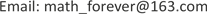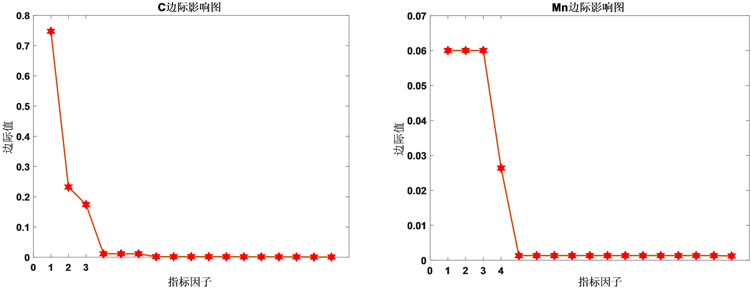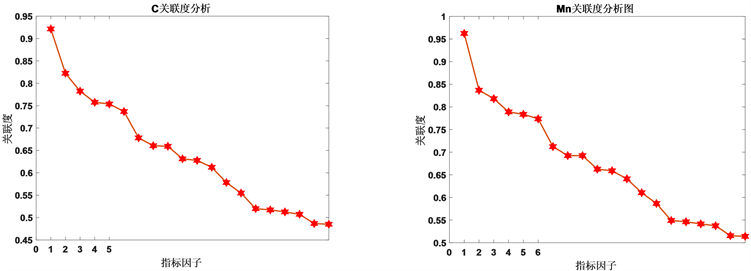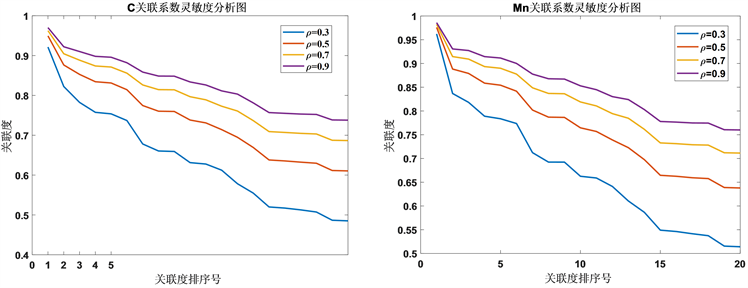﻿ 基于灰色关联度分析对合金收得率的研究 Research on Alloy Yield Based on Grey Relational Degree Analysis

Vol. 08  No. 06 ( 2019 ), Article ID: 30827 , 6 pages
10.12677/AAM.2019.86127

Research on Alloy Yield Based on Grey Relational Degree Analysis

Bixuan Li1, Kexin Zhu1, Zhuohui Zhong1, Luming Shen1,2

1College of Information Science and Technology, Hunan Agricultural University, Changsha Hunan

2Agricultural Mathematical Model and Data Process Center, Hunan Agricultural University, Changsha HunanReceived: May 25th, 2019; accepted: Jun. 12th, 2019; published: Jun. 19th, 2019ABSTRACT

In this paper, the end-point temperature of converter, the end-point metal content of converter, the metal content of normal sample in continuous casting and the quality of added materials during deoxidization and alloying are selected as the research objects. The calculation formulas of alloy yield are defined and the yield of samples in different environments is calculated, and the difference of yield among different steel species is analyzed. Then the marginal influence model is used to analyze the influence of molten steel weight and added alloy weight on the yield. Then the grey correlation model is established to investigate whether other indices which are not involved in the calculation will affect the yield of elements. The order is based on the correlation degree of each index sequence and the yield sequence of elements. The results show that the main factors affecting the yield of carbon and manganese are not significantly different, and the order of degree is temperature, silicon carbide, petroleum carburant, the proportion of S and Mn at the end of converter.

Keywords:Alloy Yield, Marginal Impact Model, Grey Relevance Degree Model

1湖南农业大学信息科学技术学院，湖南 长沙

2湖南农业大学农业数学建模与数据处理中心，湖南 长沙1. 引言

2. 数据预处理

2.1. 数据来源于模型假设

1) 在脱氧合金化的过程中，不考虑化学反应造成钢水质量的损失。

2) 元素守恒，由于在反应的过程中空气中氧气的影响，质量可以不守恒。

3) 脱氧合金化过程的温度以转炉终点的温度为标准。

2.2. 合金收得率的计算于不同钢种之间差异性检验

$Y=\frac{{C}_{1}\left({W}_{s}+\sum {W}_{i}\right)-{C}_{0}{W}_{s}}{\sum {W}_{i}{\delta }_{i}}$

3. 边际影响模型

$\frac{\partial Y}{\partial {W}_{s}}=\frac{\left({C}_{1}-{C}_{0}\right)}{\sum {W}_{i}{\delta }_{i}}$

$\frac{\partial Y}{\partial {W}_{i}}=\frac{{C}_{1}\sum {W}_{i}{\delta }_{i}-\left(\left({C}_{1}-{C}_{0}\right){W}_{s}+{C}_{1}\sum {W}_{i}\right){\delta }_{i}}{{\sum {W}_{i}{\delta }_{i}}^{\text{​}}}$Figure 1. Marginal influence diagram of indicators on carbon (left) and manganese (right) element yieldsTable 2. Ranking table of marginal impact of factors affecting carbon and manganese

4. 灰色关联度模型

${Q}_{o}=\left\{{Q}_{o}\left(k\right)|k=1,2,\cdots ,n\right\}=\left({Q}_{o}\left(1\right),{Q}_{o}\left(2\right),\cdots ,{Q}_{o}\left(n\right)\right)$

${Q}_{j}=\left\{{Q}_{j}\left(k\right)|k=1,2,\cdots ,n\right\}=\left({Q}_{j}\left(1\right),{Q}_{j}\left(2\right),\cdots ,{Q}_{j}\left(n\right)\right),j=1,2,\cdots ,23$

${r}_{i}\left(k\right)=\frac{\underset{s}{\mathrm{min}}\underset{t}{\mathrm{min}}|{Q}_{0}\left(t\right)-{Q}_{s}\left(t\right)|+\rho \underset{s}{\mathrm{max}}\underset{t}{\mathrm{max}}|{Q}_{0}\left(t\right)-{Q}_{s}\left(t\right)|}{|{Q}_{0}\left(t\right)-{Q}_{i}\left(t\right)|+\rho \underset{s}{\mathrm{max}}\underset{t}{\mathrm{max}}|{Q}_{0}\left(t\right)-{Q}_{s}\left(t\right)|}$

$Co{r}_{i}=\frac{1}{n}\underset{k=1}{\overset{n}{\sum }}{r}_{i}\left(k\right)$Figure 2. Relevance analysis of carbon (left) and manganese (right) elementsTable 3. Ranking of correlation degree of influencing factors of carbon and manganese recovery

5. 灰色关联度模型的灵敏度检验

6. 结语Figure 3. Sensitivity analysis diagram based on correlation coefficient

Research on Alloy Yield Based on Grey Relational Degree Analysis[J]. 应用数学进展, 2019, 08(06): 1101-1106. https://doi.org/10.12677/AAM.2019.86127

1. 1. 陈襄武. 炼钢过程的脱氧[M]. 北京: 冶金工业出版社, 1991.

2. 2. 李丰功, 战东平, 张佩灿, 等. 脱氧合金化工艺对高铝钢中夹杂物的影响[J]. 中国冶金, 2013, 23(7): 21-23.

3. 3. 林鹏程, 吴启勋. 灰色关联度分析及应用[J]. 盐湖研究, 2001, 9(2): 48-50.

4. 4. 杨怀春, 马进国, 姜国才. 碳化硅对转炉炼钢脱氧合金化的影响分析[J]. 新疆钢铁, 2014(2): 43-45.